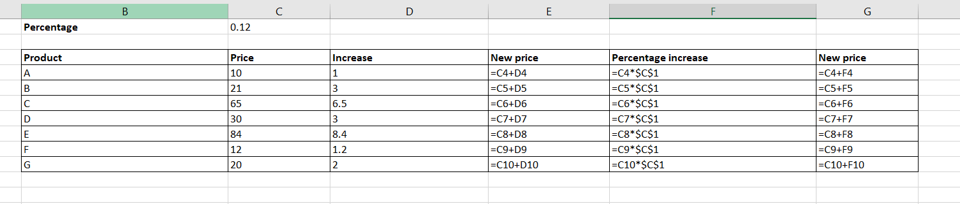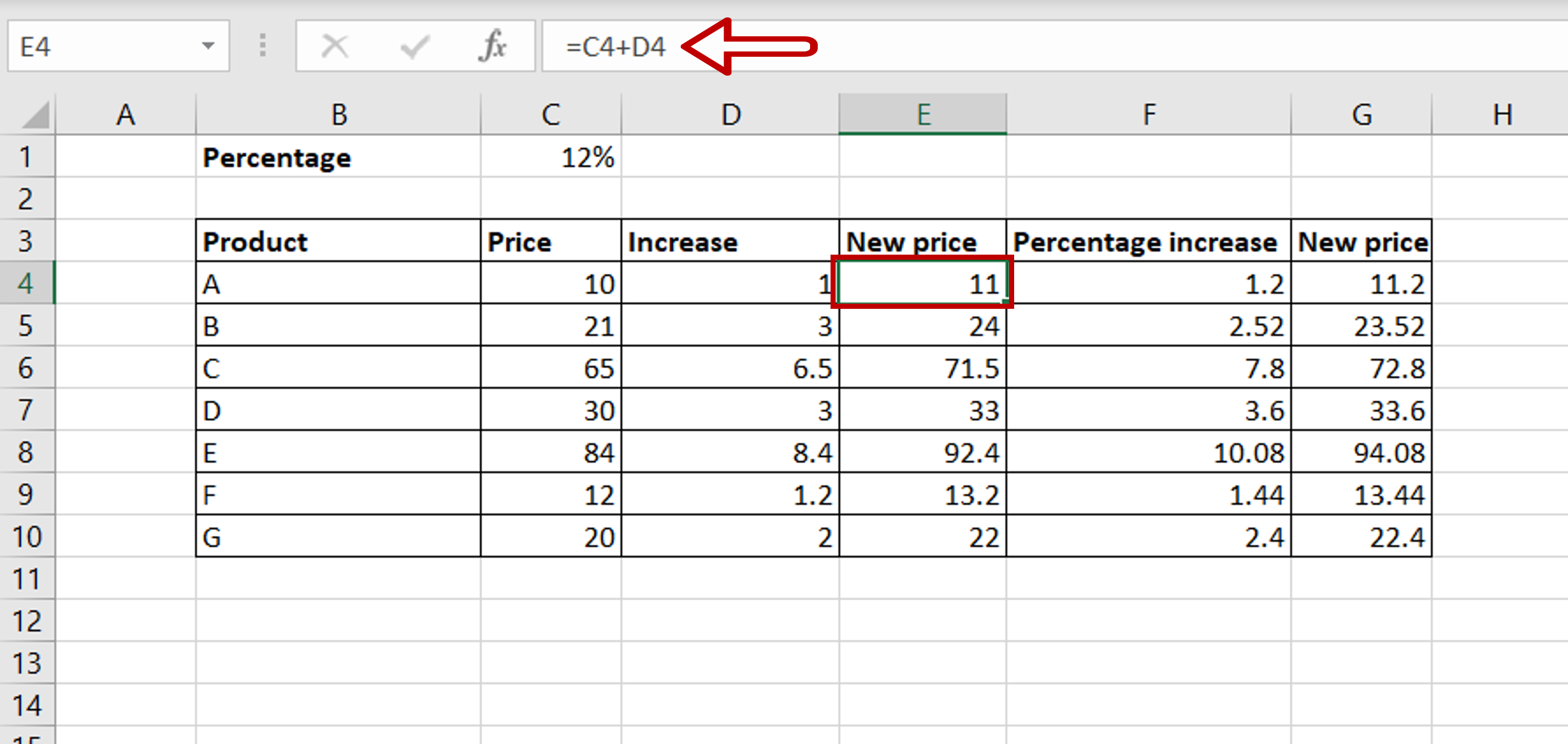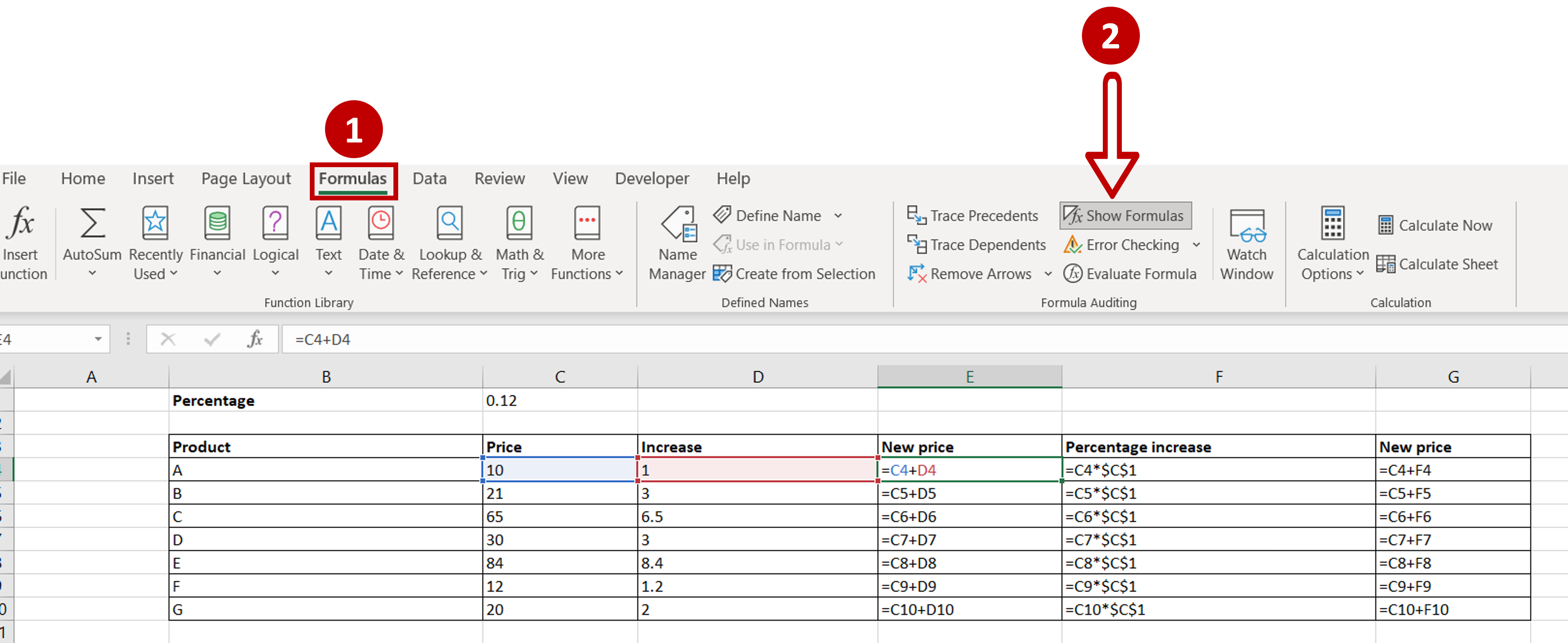# How do you display formulas in Excel

You can watch a video tutorial here.Excel is widely used for calculations and can be used to create complex formulas. When studying an Excel sheet with a lot of columns that have calculated values, it is useful to see the formulas to understand how the calculations have been done.

### Step 1 – Check if the cells have formulas– Select a cell
– Check the Formula bar to see if it contains a formula

### Step 2 – Show the formulas– Go to Formulas > Formula Auditing
– Click on Show Formulas

### Step 3 – Check the result– Formulas are displayed in those cells that contain formulas